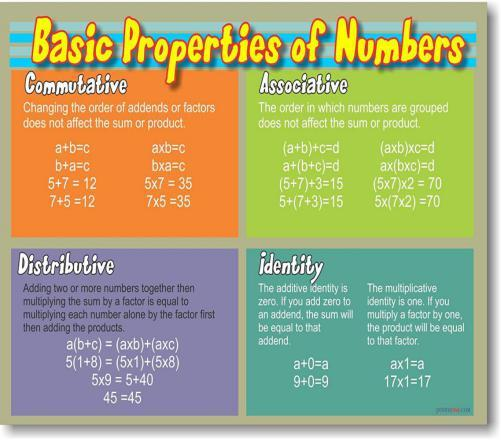# What Are The Properties Of Numbers?

Approved & Edited by ProProfs Editorial Team
The editorial team at ProProfs Quizzes consists of a select group of subject experts, trivia writers, and quiz masters who have authored over 10,000 quizzes taken by more than 100 million users. This team includes our in-house seasoned quiz moderators and subject matter experts. Our editorial experts, spread across the world, are rigorously trained using our comprehensive guidelines to ensure that you receive the highest quality quizzes.
| By Jaksiboy
J
Jaksiboy
Community Contributor
Quizzes Created: 919 | Total Attempts: 12,132,600
Questions: 10 | Attempts: 168SettingsA number is a mathematical object used to count, measure, and label. What more things do you know about the number? Come on take this quiz and have fun.

• 1.

### Which of the following is known as a notation that represents a number?

• A.

Numerals

• B.

Digits

• C.

Class

• D.

Objects

A. Numerals
Explanation
Numerals are a notation system used to represent numbers. They are symbols or characters that are used to express numerical quantities. In contrast, digits refer to the individual symbols used in numeral systems, such as 0, 1, 2, etc. Class and objects are not directly related to representing numbers.

Rate this question:

• 2.

### A=b. which of the following best describes the equation above?

• A.

Reflexive property

• B.

Symmetry property

• C.

Commutative property

• D.

None of the above

A. Reflexive property
Explanation
The equation "a=b" represents the reflexive property because it states that "a" is equal to "b", which means that "a" is equal to itself. The reflexive property states that any element is equal to itself, so this equation satisfies that property.

Rate this question:

• 3.

### If a+b=c, then c= a+b. Which of the following best describes the above equation?

• A.

Reflexive

• B.

Symmetric

• C.

Commutative

• D.

Transitive

B. Symmetric
Explanation
The given equation states that if a+b equals c, then c equals a+b. This implies that the order of the terms does not matter. If a is added to b, it will always result in the same value, regardless of the order in which they are added. This property is known as symmetry, making "Symmetric" the best description for the equation.

Rate this question:

• 4.

### If a=b,b=c then a=c. Which of the following best describes the equation above?

• A.

Symmetry

• B.

Reflexive

• C.

Transitive

• D.

Commutative

C. Transitive
Explanation
The given equation states that if a is equal to b, and b is equal to c, then a must also be equal to c. This is the definition of transitivity, where a relationship between three elements holds true if it holds true for any two pairs of those elements.

Rate this question:

• 5.

### A*b=b*a. which of the following equations best descibes the above equation?

• A.

Commutative

• B.

Transitive

• C.

All of the above

• D.

None of the above

A. Commutative
Explanation
The equation a*b=b*a is an example of the commutative property of multiplication. This property states that the order of the numbers being multiplied does not affect the result. In other words, when multiplying two numbers, you can swap their positions and still get the same answer. Therefore, the correct answer is "Commutative."

Rate this question:

• 6.

### Which of the following notation represents a natural number?

• A.

A

• B.

N

• C.

Z

• D.

R

B. N
Explanation
The notation "N" represents a natural number. Natural numbers are the set of positive integers starting from 1 and extending to infinity. The letter "N" is commonly used to denote this set in mathematics.

Rate this question:

• 7.

### Which of the following notation represent a complex number?

• A.

A

• B.

Z

• C.

C

• D.

N

C. C
Explanation
The notation "C" represents a complex number. Complex numbers are numbers that can be expressed in the form a + bi, where "a" and "b" are real numbers and "i" is the imaginary unit. The "C" notation is commonly used in mathematics to denote the set of complex numbers.

Rate this question:

• 8.

### Which of the following represents a real number?

• A.

A

• B.

Z

• C.

N

• D.

None of the number

D. None of the number
Explanation
The options provided - A, Z, and N - do not represent real numbers. A represents the set of natural numbers, Z represents the set of integers, and N represents the set of whole numbers. Real numbers include rational and irrational numbers, such as fractions, decimals, and square roots of non-perfect squares. Therefore, none of the given options represent a real number.

Rate this question:

• 9.

### Which of the following is an example of  real number?

• A.

12

• B.

12.0

• C.

-12

• D.

None of the abpve

B. 12.0
Explanation
The number 12.0 is an example of a real number because it is a decimal number that can be expressed as a finite or infinite decimal. Real numbers include rational numbers (numbers that can be expressed as a fraction) and irrational numbers (numbers that cannot be expressed as a fraction and have an infinite decimal representation). Since 12.0 can be expressed as the fraction 12/1, it is a rational number and therefore a real number.

Rate this question:

• 10.

### Which of the following is an example of even numbers?

• A.

1

• B.

13

• C.

14

• D.

17

C. 14
Explanation
An even number is a number that is divisible by 2 without leaving a remainder. In this case, the number 14 is the only option that is divisible by 2, as 14 divided by 2 equals 7 with no remainder. Therefore, 14 is an example of an even number.

Rate this question:

Related TopicsBack to top